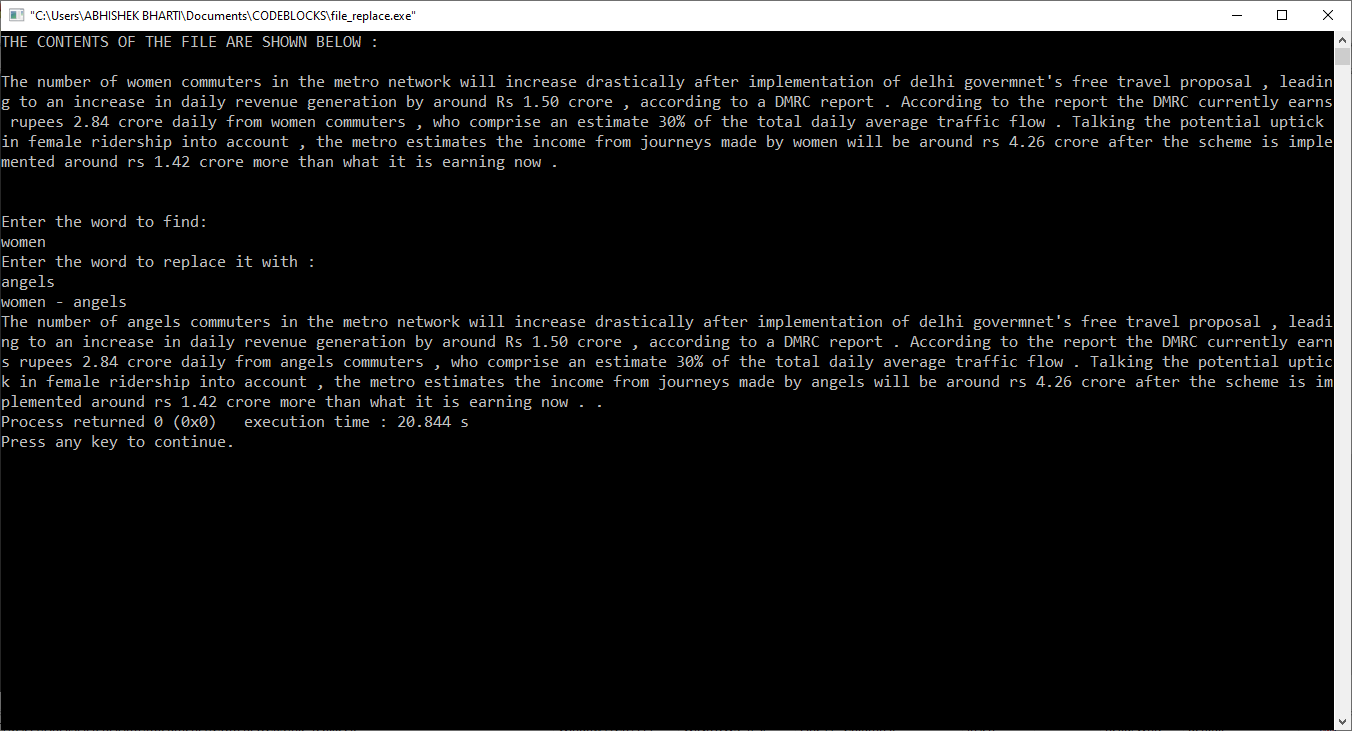Open In App

# C program to find and replace a word in a File by another given word

Pre-requisite: File Handling in C
Given a file containing some text, and two strings wordToBeFind and wordToBeReplacedWith, the task is to find all occurrences of the given word ‘wordToBeFind’ in the file and replace them with the given word ‘wordToBeReplacedWith’.

Examples:

```Input : File = "xxforxx xx for xx",
wordToBeFind = "xx",
wordToBeReplacedWith = "geeks"
Output : geeksforgeeks geeks for geeks```

Approach: The idea here is to read the contents from the given file, process the find and replace, and then store the output in another file.

1. Make objects of FILE (ifp and ofp)
2. Open two files, one for file input in read mode and another in write+ mode
3. Check for the file to be opened correctly
4. Read the contents of the existing input file word by word
5. As using fgets takes the input of new line character(i.e. enter key) also we just copy the null character of the string one position back so that the newline is replaced with “\0”
6. We run a loop till the end of file is reached and scan each word in the file and store it in a variable “read”.
7. Then we compare “read” with “wordToBeFind” and if the result is true, we use “strcpy()” to replace “read” with “wordToBeReplacedWith”.
8. Show the word replacement through printf
9. Now again we shift the filepointer at the beginning of the file and print the file contents of the output file.

Below is the implementation of the above approach:

## C

 `// C program to find and replace a word``// in a File by another given word` `#include ``#include ``#include ` `// Function to find and``// replace a word in File``void` `findAndReplaceInFile()``{``    ``FILE` `*ifp, *ofp;``    ``char` `word, ch, read, replace;``    ``int` `word_len, i, p = 0;` `    ``ifp = ``fopen``(``"file_search_input.txt"``, ``"r"``);``    ``ofp = ``fopen``(``"file_replace_output.txt"``, ``"w+"``);``    ``if` `(ifp == NULL || ofp == NULL) {``        ``printf``(``"Can't open file."``);``        ``exit``(0);``    ``}``    ``puts``(``"THE CONTENTS OF THE FILE ARE SHOWN BELOW :\n"``);` `    ``// displaying file contents``    ``while` `(1) {``        ``ch = ``fgetc``(ifp);``        ``if` `(ch == EOF) {``            ``break``;``        ``}``        ``printf``(``"%c"``, ch);``    ``}` `    ``puts``(``"\n\nEnter the word to find:"``);``    ``fgets``(word, 100, stdin);` `    ``// removes the newline character from the string``    ``word[``strlen``(word) - 1] = word[``strlen``(word)];` `    ``puts``(``"Enter the word to replace it with :"``);``    ``fgets``(replace, 100, stdin);` `    ``// removes the newline character from the string``    ``replace[``strlen``(replace) - 1] = replace[``strlen``(replace)];` `    ``fprintf``(ofp, ``"%s - %s\n"``, word, replace);` `    ``// comparing word with file``    ``rewind``(ifp);``    ``while` `(!``feof``(ifp)) {` `        ``fscanf``(ifp, ``"%s"``, read);` `        ``if` `(``strcmp``(read, word) == 0) {` `            ``// for deleting the word``            ``strcpy``(read, replace);``        ``}` `        ``// In last loop it runs twice``        ``fprintf``(ofp, ``"%s "``, read);``    ``}` `    ``// Printing the content of the Output file``    ``rewind``(ofp);``    ``while` `(1) {``        ``ch = ``fgetc``(ofp);``        ``if` `(ch == EOF) {``            ``break``;``        ``}``        ``printf``(``"%c"``, ch);``    ``}` `    ``fclose``(ifp);``    ``fclose``(ofp);``}` `// Driver code``void` `main()``{``    ``findAndReplaceInFile();``}`

How to execute the above code:

1. Copy the source code from here and paste it in an offline IDE
2. Save the program.
3. Create a file named “file_search_input.txt” and save it in the folder where you saved the above-copied program.
4. Now open the terminal or offline IDE and run the program

Output:Time complexity: O(n)

Where n is the number of characters in the file being searched. This is because the time taken for this algorithm is directly proportional to the number of characters in the file.

Space complexity: O(1)

This algorithm does not use any additional data structures and hence the space complexity is constant, O(1).